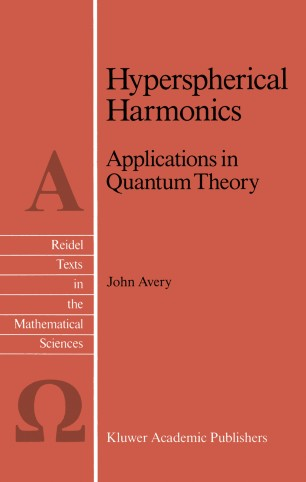# Hyperspherical Harmonics

## Applications in Quantum Theory

• John AveryBook

Part of the Reidel Texts in the Mathematical Sciences book series (RTMS, volume 5)

1. Front Matter
Pages i-xvi
2. John Avery
Pages 1-10
3. John Avery
Pages 11-24
4. John Avery
Pages 25-46
5. John Avery
Pages 47-57
6. John Avery
Pages 59-76
7. John Avery
Pages 77-92
8. John Avery
Pages 105-125
9. John Avery
Pages 127-139
10. John Avery
Pages 141-173
11. John Avery
Pages 175-188
12. Back Matter
Pages 189-255

### Introduction

where d 3 3)2 ( L x - -- i3x j3x j i i>j Thus the Gegenbauer polynomials play a role in the theory of hyper spherical harmonics which is analogous to the role played by Legendre polynomials in the familiar theory of 3-dimensional spherical harmonics; and when d = 3, the Gegenbauer polynomials reduce to Legendre polynomials. The familiar sum rule, in 'lrlhich a sum of spherical harmonics is expressed as a Legendre polynomial, also has a d-dimensional generalization, in which a sum of hyper spherical harmonics is expressed as a Gegenbauer polynomial (equation (3-27»: The hyper spherical harmonics which appear in this sum rule are eigenfunctions of the generalized angular monentum 2 operator A , chosen in such a way as to fulfil the orthonormality relation: VIe are all familiar with the fact that a plane wave can be expanded in terms of spherical Bessel functions and either Legendre polynomials or spherical harmonics in a 3-dimensional space. Similarly, one finds that a d-dimensional plane wave can be expanded in terms of HYPERSPHERICAL HARMONICS xii "hyperspherical Bessel functions" and either Gegenbauer polynomials or else hyperspherical harmonics (equations ( 4 - 27) and ( 4 - 30) ) : 00 ik·x e = (d-4)!!A~oiA(d+2A-2)j~(kr)C~(~k'~) 00 (d-2)!!I(0) 2: iAj~(kr) 2:Y~ (["2k)Y (["2) A A=O ). l). l)J where I(O) is the total solid angle. This expansion of a d-dimensional plane wave is useful when we wish to calculate Fourier transforms in a d-dimensional space.

### Keywords

Atom atoms hydrogen quantum theory symmetry wave

#### Authors and affiliations

• John Avery
• 1
1. 1.Department of Physical Chemistry, H.C. Ørsted InstituteUniversity of CopenhagenDenmark

### Bibliographic information

• DOI https://doi.org/10.1007/978-94-009-2323-2